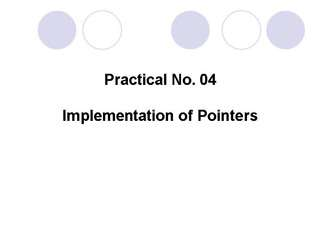# Pointer Implemetation - PowerPoint PPT PresentationDownload PresentationPointer Implemetation

Pointer Implemetation
Download Presentation## Pointer Implemetation

- - - - - - - - - - - - - - - - - - - - - - - - - - - E N D - - - - - - - - - - - - - - - - - - - - - - - - - - -
##### Presentation Transcript

1. Practical No. 04Implementation of Pointers

2. Pointers • Pointers are variables that contain memoryaddresses as their values. • A variable name directly references a value. • A pointer indirectly references a value. Referencing a value through a pointer is called indirection. • A pointer variable must be declared before it can be used.

4. Consider the statements: #include <stdio.h> int main ( ) { FILE *fptr1 , *fptr2 ; /* Declare two file pointers */ int *aptr ; /* Declare a pointer to an int */ float *bptr ; /* Declare a pointer to a float */ int a ; /* Declare an int variable */ float b ; /* Declare a float variable */

5. Use of & and * • When is & used? • When is * used? • & -- "address operator" which gives or produces the memory address of a data variable • * -- "dereferencing operator" which provides the contents in the memory location specified by a pointer

6. Pointers and Functions • Pointers can be used to pass addresses of variables to called functions, thus allowing the called function to alter the values stored there. • If in a function the values stored in the main program do not change when they are passed to another function then This is known as "call by value".

7. Pointers and Functions • If instead of passing the values of the variables to the called function, we pass their addresses, so that the called function can change the values stored in the calling routine. This is known as "call by reference" since we are referencing the variables. • The following shows the swap function as a "call by reference". Note that the values are now actually swapped when the control is returned to main function.

8. Pointers with Functions (example) void swap( int *a, int *b ) { int temp; temp= *a; *a= *b; *b = temp ; cout<<"a=“<<a<<“b=“<<b; } Results: a=5 b=6 a=6 b=5 a=6 b=5 #include <constream.h> void swap ( int *a, int *b ) ; int main ( ) { int a = 5, b = 6; cout<<"a=“<<a<<“b=“<<b; swap (&a, &b) ; cout<<"a=“<<a<<“b=“<<b; }

9. Arithmetic and Logical Operations on Pointers • A pointer may be incremented or decremented • An integer may be added to or subtracted from a pointer. • Pointer variables may be subtracted from one another.

10. Arithmetic Operations on Pointers • When an integer is added to or subtracted from a pointer, the new pointer value is changed by the integer times the number of bytes in the data variable the pointer is pointing to. • For example, if the pointer valptr contains the address of a double precision variable and that address is 234567870, then the statement: valptr = valptr + 2; would change valptr to 234567886

11. Using the C++ Language Special Keyword sizeof • This keyword can be used to determine the number of bytes in a data type, a variable, or an array • Example: double array ; sizeof (double); /* Returns the value 8 */ sizeof (array); /* Returns the value 80 */ sizeof(array)/sizeof(double); /* Returns 10 */